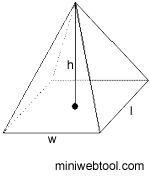×

# Volume of a Pyramid CalculatorPyramid base width (w): Pyramid base length (l): Pyramid base height (h):

## About Volume of a Pyramid Calculator

The Volume of a Pyramid Calculator is used to help you find the volume of a pyramid.

## Volume of a Pyramid Formula

The following is the calculation formula for the volume of a pyramid:

V = lwh/3

Where:
V = volume of a pyramid
l = pyramid base length
w = pyramid base width
h = pyramid base height

## All of Our Miniwebtools (Sorted by Name):

•{{name}}
{{title}}

Miniwebtool

If you like Volume of a Pyramid Calculator, please consider adding a link to this tool by copy/paste the following code:

Watch Videos: